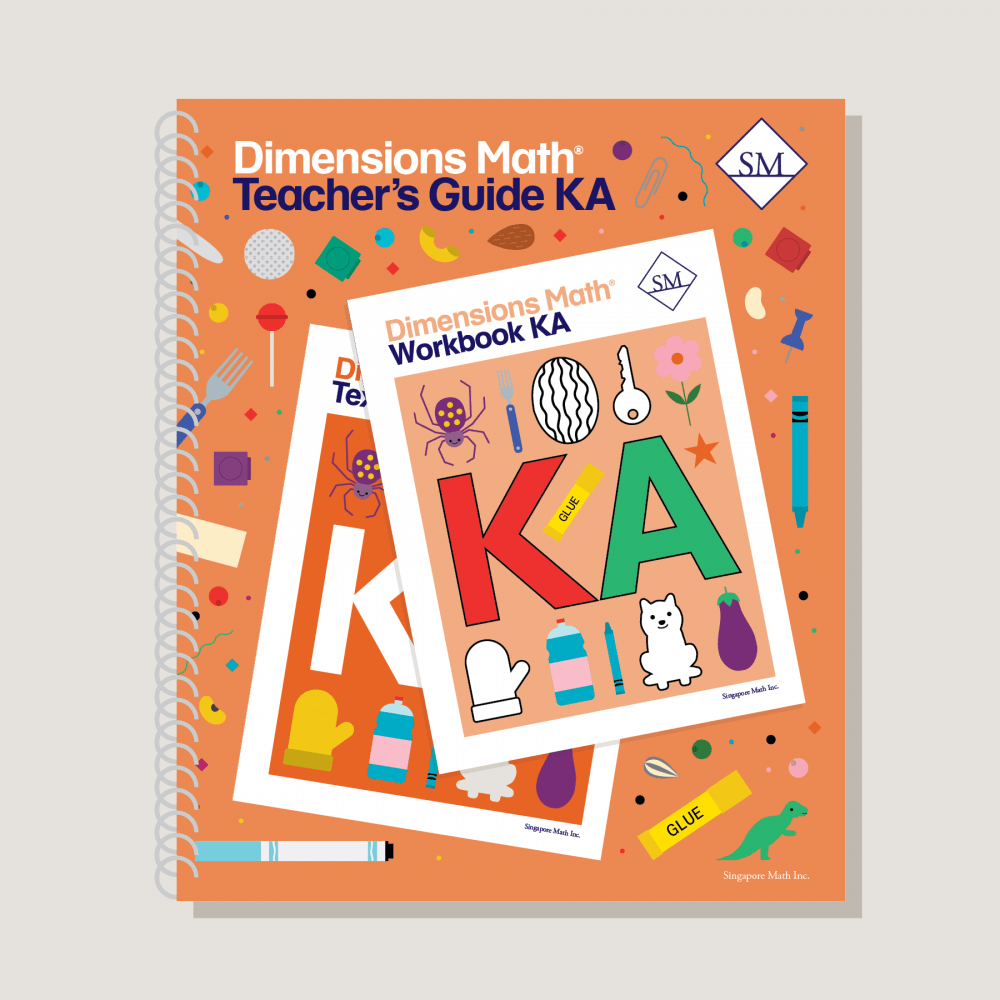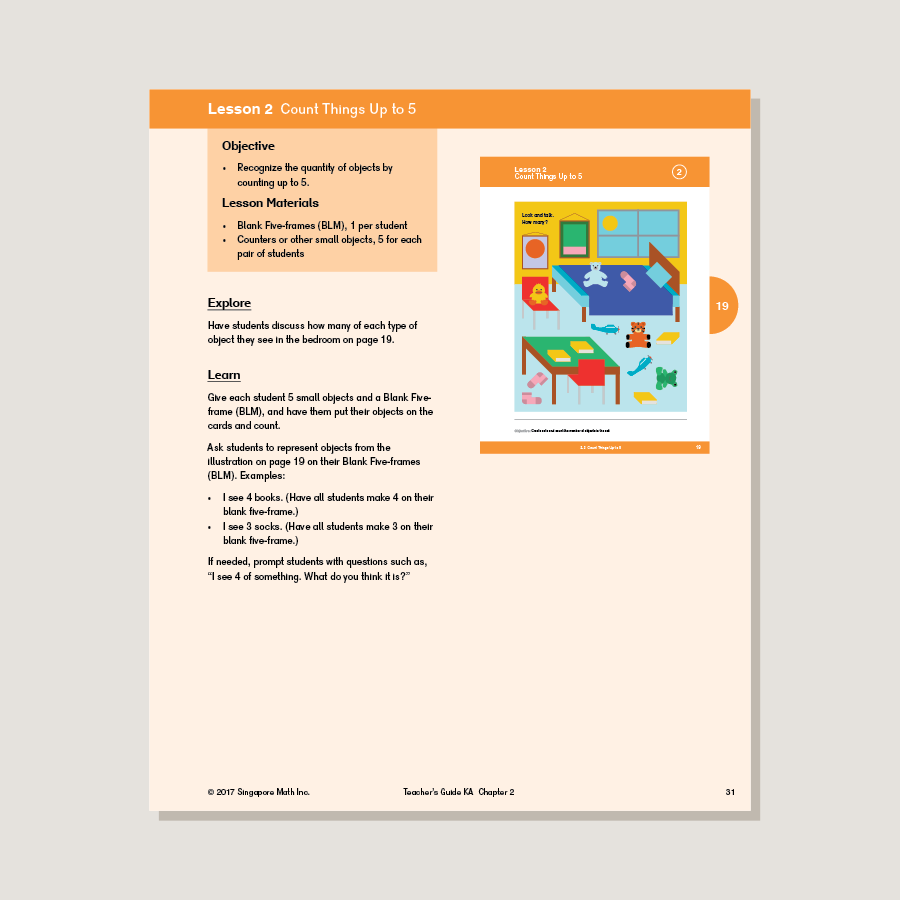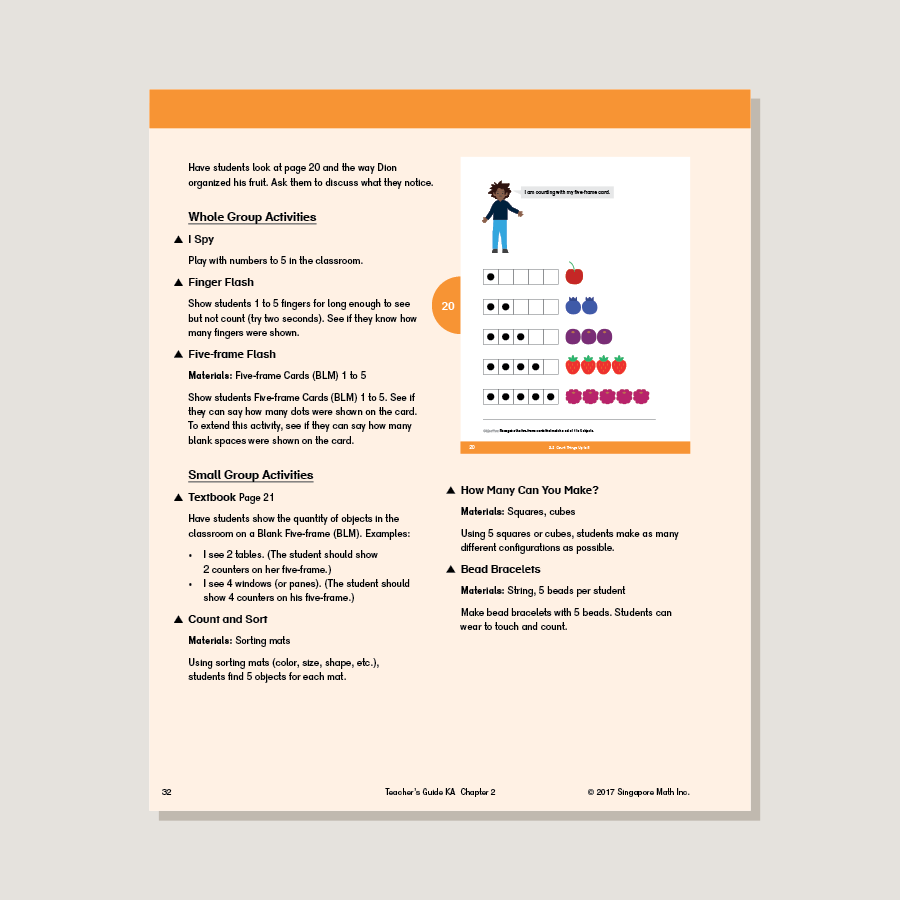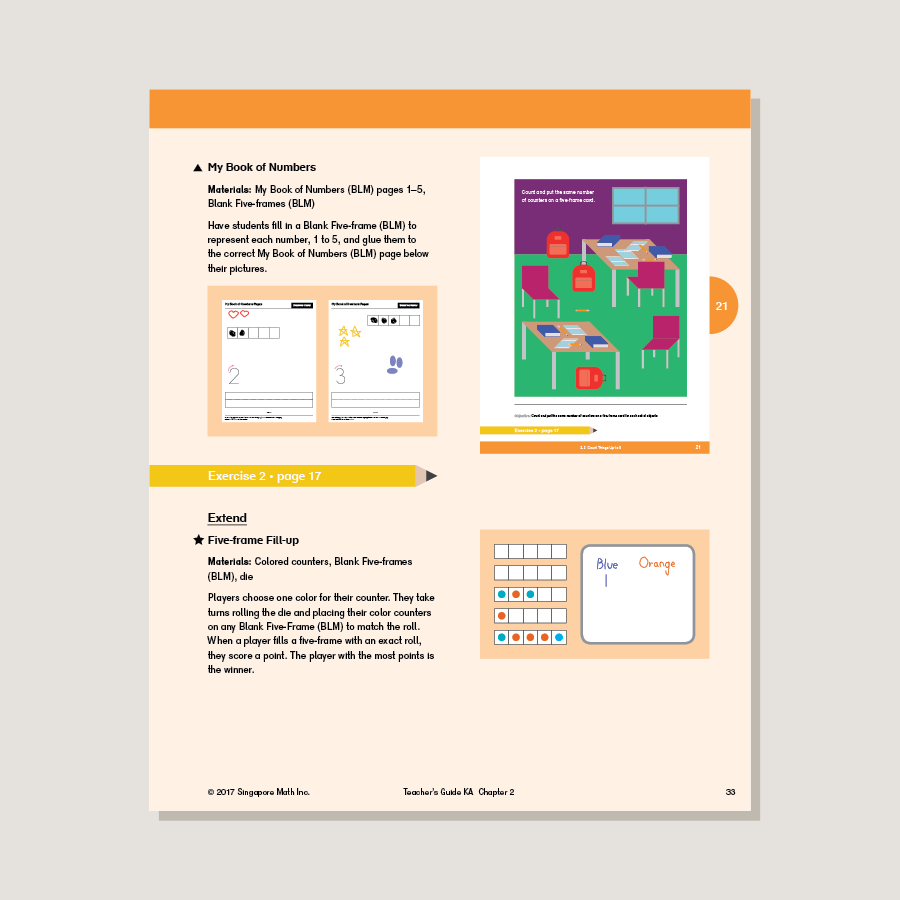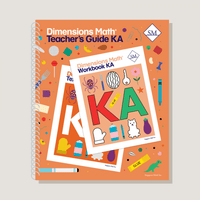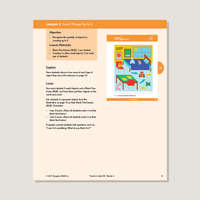# Dimensions Math Teacher's Guide KA

\$29.50

Teacher’s guides are a comprehensive resource for achieving lesson objectives. They include background information, detailed lesson plans, helpful suggestions, and activities. They help educators understand the purpose of each lesson within the context of the curriculum. A high level of educator involvement is essential for success in this program.

Note: Hard cover. Spiral-bound. Manipulative Kits (sold separately) include all the essential manipulatives used in Teacher’s Guides activities.

SKU: DMTGKA

ISBN: 9781947226302

Pagecount: 218

Dimensions: 11 x 12 x 1 in

Weight: 3.15 lbs

Binding: Spiral

Color: Color

Cover: Hard

Perforated: No

Sample Pages

Chapter 1: Match, Sort, and Classify
Teaching Notes
Chapter Opener
Lesson 1: Left and Right
Lesson 2: Same and Similar
Lesson 3: Look for One that is Different
Lesson 4: How Does it Feel?
Lesson 5: Match the Things That Go Together
Lesson 6: Sort
Workbook Solutions

Chapter 2: Numbers to 5
Teaching Notes
Chapter Opener
Lesson 1: Count to 5
Lesson 2: Count Things up to 5
Lesson 3: Recognize the Numbers 1 to 3
Lesson 4: Recognize the Numbers 4 and 5
Lesson 5: Count and Match
Lesson 6: Write the Numbers 1 and 2
Lesson 7: Write the Number 3
Lesson 8: Write the Number 4
Lesson 9: Trace and Write 1 to 5
Lesson 10: Zero
Lesson 11: Picture Graphs
Lesson 12: Practice
Workbook Solutions

Chapter 3: Numbers to 10
Teaching Notes
Chapter Opener
Lesson 1: Count 1 to 10
Lesson 2: Count Up to 7 Things
Lesson 3: Count Up to 9 Things
Lesson 4: Count Up to 10 Things — Part 1
Lesson 5: Count Up to 10 Things — Part 2
Lesson 6: Recognize the Numbers 6 to 10
Lesson 7: Write the Numbers 6 and 7
Lesson 8: Write the Numbers 8, 9, and 10
Lesson 9: Write the Numbers 6 to 10
Lesson 10: Count and Write the Numbers 1 to 10
Lesson 11: Ordinal Positions
Lesson 12: One More Than
Lesson 13: Practice
Workbook Solutions

Chapter 4: Shapes and Solids
Teaching Notes
Chapter Opener
Lesson 1: Curved or Flat
Lesson 2: Solid Shapes
Lesson 3: Closed Shapes
Lesson 4: Rectangles
Lesson 5: Squares
Lesson 6: Circles and Triangles
Lesson 7: Where is It?
Lesson 8: Hexagons
Lesson 9: Sizes and Shapes
Lesson 10: Combine Shapes
Lesson 11: Graphs
Lesson 12: Practice
Workbook Solutions

Chapter 5: Compare Height, Length, Weight, and Capacity
Teaching Notes
Chapter Opener
Lesson 1: Comparing Height
Lesson 2: Comparing Length
Lesson 3: Height and Length — Part 1
Lesson 4: Height and Length — Part 2
Lesson 5: Weight — Part 1
Lesson 6: Weight — Part 2
Lesson 7: Weight — Part 3
Lesson 8: Capacity — Part 1
Lesson 9: Capacity — Part 2
Lesson 10: Practice
Workbook Solutions

Chapter 6: Comparing Numbers Within 10
Teaching Notes
Chapter Opener
Lesson 1: Same and More
Lesson 2: More and Fewer
Lesson 3: More and Less
Lesson 4: Practice — Part 1
Lesson 5: Practice — Part 2
Workbook Solutions

Blackline Masters for KA

A & B Books: Our programs divide the school year into two semesters. “A” level books are for the first half of the school year. “B” level books are for the second half of the school year. You need both “A” and “B” material for a complete school year.

Required Components: Textbooks, Workbooks, and Guides (either Home Instructor’s Guides or Teacher’s Guides) are all necessary components. These three elements each serve a unique function and work together to build math mastery.

Printouts: Dimensions Math PK-5 Resources

Recommended Manipulatives: Dimensions Math Grades PK-5 Recommended Manipulatives

Answer Key: Answers included for corresponding Textbook and Workbook

Dimensions Math At Home™ Videos

Invite a professional Singapore math teacher into your home classroom. This subscription of pre-recorded lessons covers all Textbook and Workbook material for an entire school year and can be done at your own pace.

Manipulatives

Manipulatives help students visualize and represent math concepts. Stock up on suggested items to deepen engagement during math activities and lessons.

Supplementary Math

Supplementary math helps students of all abilities: struggling, advanced, or on level. Find just the right addition to round out your core program.